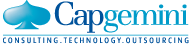# Placement Papers - CapgeminiCapgemini Interview Experience - RCCIIT, Kolkata on 21/09/2017
Posted by :
Abhishek Pal
(259)
Hello friends, here is the total deal of Capgemini:-

Round 1 - Aptitude Test (16 Quants + 16 Reasoning) Total time- 25+25=50 mins. There is no hard and fast rule that you have to complete 1 section first and then you can switch over to the other one.

Capgemini sets a sectional cut-off as well as an aggregate cut-off, both of which are kept confidential as a deal is struck between the company and the corresponding college.

Then comes Essay writing. 30 mins is allotted for this round. Min 200 words, Max 240 words. Ample amount of time and you can easily complete the essay within the stipulated time.

Round 2- Pseudocode.
20 MCQs,20 mins time.
All the question paper sets of each and every round were different.

Then comes PI and HR on the 2nd day(22/09/2017).

I could not clear the aptitude round but I would like to help others with the questions that I can remember.

Round 1- Quants:

1) Find out the average of a,b,c,d,e,f.

2) If A can complete a piece of work in 12 days and B can complete the same work in 16 days and if A+B+C can complete the work in 4 days, find out in how many days can C complete the work.

3) Average speed numerical using formula (2*x*y)/(x+y) [2 such sums].

4) Find the no. of diagonals of a polygon having 16 sides.

5) Sum on Expenditure and Income and Savings.

6) Numbers marked from 1-20.Find the probability of choosing a no. which is a multiple of 3.

7) Total amount is given 13200. If A gets 2/3 rd of B\'s share.B gets 1/4th of C\'s share. Find out B\'s share.

8)Problem based on log. Something like: Simplify Log a+Log b+ ...... Log 20.

9)Function based problem. f()= something h()= something g()= something. Evaluate fohog.

10)Find the 1/3rd of 3333.

11)Current ages of Father and son is in a ratio. After 5 years their ration is (something).What is the current age of the son?

Rest were from PROFIT AND LOSS,TRAINS AND PLATFORMS, INVESTMENT.

Reasoning:

1) A flowchart was given regarding recruitment of a candidate.
Question A: In total how many times the candidate is liable to rejection?
Question B: What is the 2nd last round before the candidate can get his offer letter?

2) A Data Graph was given comprising of Income and one more Variable.
Question A: Calculate the % of A-B on A
Question B: again related to %

3) If A@B means something (not greater)
A#B means something (not equal)
A&B means something
Evaluate P#Q@S&R ... and so on and so forth.

4) If A@B means A is the father of B
A#B means A is the daughter of B
A&B means A is the grand son of B
Evaluate P#Q@S&R ... and so on and so forth.

5) Paper cutting sum.

6) Problem figure.

7) Syllogism(2-3 such questions).

8) Coding-Decoding.

9) Find the no. of 4\'s that are preceded by # and succeeded by @ in a particular expression.

10) Data sufficiency question.

11) An expression is given followed by 4 options marked as A,B,C,D.Find out the option which weakens the corresponding expression.

Rest were from MISSING CHARACTER,VERIFICATION OF TRUTH.

Essay :

Topics- 1) Good intentions are not valuable if they are not converted into concrete action.

2) Something related to Ethics and moral principles.
etc.

Pseudocode round questions.. as told by the qualified students:-

Out of 20 MCQs 16 were from C i.e Find the output,2 from Data Structure,2 from Digital Electronics.

In some cases 8 from C,2 from Digital Electronics,6 from DS,4 from basic programming.

I have no idea regarding PI and HR rounds.

I would like to suggest certain points.

1) Sectional cut-off plays a big factor in Apti round so keep it in mind.

2) Essay writing- Space after(.) Space after(,) Minimum mistakes in spellings and avoid using backspace.

Good Luck :).